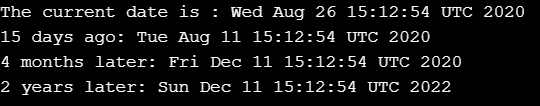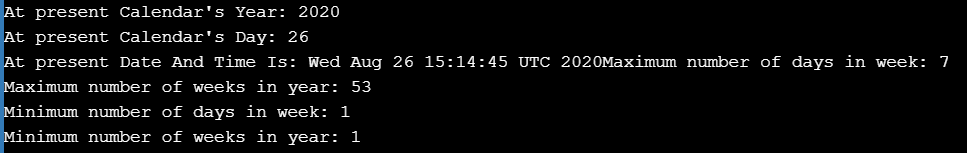# Java Calendar Class

## Java Calendar Class with Examples

In this article, I am going to discuss Java Calendar Class with Examples. Please read our previous article where we discussed Date and Time API in Java.

##### Java Calendar Class:

java.util.Calendar is an abstract class. It provides methods for converting date between a specific instant in time and a set of calendar fields such as MONTH, YEAR, HOUR, etc. We cannot use a constructor to create an instance because it is an Abstract class. Therefore, we have to use the static method Calendar.getInstance() to instantiate and implement a sub-class.

Calendar.getInstance(): It returns a Calendar instance based on the current time in the default time zone with the default locale.
Calendar.getInstance(TimeZone zone)
Calendar.getInstance(Locale aLocale)
Calendar.getInstance(TimeZone zone, Locale aLocale)

##### The important points about Calendar −

It also provides additional fields and methods for implementing a concrete calendar system outside the package. It defines the range of values returned by certain calendar fields.

Declaration: public abstract class Calendar extends Object implements Serializable, Cloneable, Comparable<Calendar>

##### Methods
1. public void add(int field, int amount): It is used to add the specified amount of time to the given calendar field.
2. public boolean after(Object when): It returns true if the time represented by this Calendar is after the time represented by when Object.
3. public boolean before(Object when): It basically returns true if the time represented by this Calendar is before the time represented by when Object.
4. public final void clear(int field): It is used to set the given calendar field value and the time value of this Calendar undefined.
5. public Object clone(): It provides the copy of the current object.
6. public int compareTo(Calendar anotherCalendar): It is used to compare the time value between two calendar objects.
7. protected void complete(): It is used to fill any unset fields in the calendar fields.
8. protected abstract void computeFields(): It is used to convert the current millisecond time value time to calendar field values in fields[].
9. protected abstract void computeTime(): It is used to convert calendar field values in the field[] to the millisecond time value time.
10. public boolean equals(Object object): It is used to compare two objects for equality and returns true if they are equal.
11. public int get(int field): It is passed as the parameter and returns the value of fields passed as the parameter.
12. public int getActualMaximum(int field): It returns the maximum possible value of the calendar field passed as the parameter to this method.
13. public int getActualMinimum(int field): It returns the minimum possible value of the calendar field passed as the parameter to this method.
14. public static Set<String> getAvailableCalendarTypes(): It returns a set which contains string set of all available calendar type supported by JRE.
15. public static Locale[] getAvailableLocales(): It returns an array of all locales available in JRE.
16. public String getCalendarType(): It returns all available calendar types supported by JRE.
17. public String getDisplayName(int field, int style, Locale locale): It returns the String representation of the calendar field value passed as the parameter in a given style and local.
18. public Map<String, Integer> getDisplayNames(int field, int style, Locale locale): It returns Map representation of the calendar field value passed as the parameter.
19. public int getFirstDayOfWeek(): It returns the first day of the week in integer form.
20. public abstract int getGreatestMinimum(int field): It returns the highest minimum value of the Calendar field passed as the parameter.
21. public static Calendar getInstance(): It is used with calendar objects to get the instance of the calendar according to the current time zone set by JRE.
22. public abstract int getLeastMaximum(int field): It returns the smallest value from all maximum value for the field specified as the parameter to the method.
23. public abstract int getMaximum(int field): It is used with calendar object to get the maximum value of the specified calendar field as the parameter.
24. public int getMinimalDaysInFirstWeek(): It returns required minimum days in integer form.
25. public abstract int getMinimum(int field): It is used with calendar objects to get the minimum value of the specified calendar field as the parameter.
26. public final Date getTime(): It gets the time value of the calendar object and returns the date.
27. public long getTimeInMillis(): It returns the current time in millisecond. Its returns type is long.
28. public TimeZone getTimeZone(): It gets the Time zone of calendar object and returns a Time zone object.
29. public int getWeeksInWeekYear(): It returns total weeks in week year. It is returned in integer form.
30. public int getWeekYear(): It gets the week year represented by current Calendar.
31. public int hashCode(): It returns the hash code for calendar object.
32. Protected final int internalGet(int field): It returns the value of the calendar field passed as the parameter.
33. public boolean isLenient(): It returns the Boolean value True if the interpretation mode of this calendar is lenient; false otherwise.
34. public final boolean isSet(int field): It will check if the specified field as the parameter has been set or not. Returns false if it is not set; otherwise true.
35. public boolean isWeekDateSupported(): It will check if this calendar supports the week date. Its default value is false.
36. public abstract void roll(int field, Boolean up): It increases or decreases the specified calendar field by one unit without affecting the other field.
37. public void set(int field, int value): It sets the specified calendar field by the specified value.
38. public void setFirstDayOfWeek(int value): It sets the first day of the week. The value to be set is passed as a parameter.
39. public void setMinimalDaysInFirstWeek(int value): It sets the minimal days required in the first week. The value to be set is passed as a parameter.
40. public final void setTime(Date date): It sets the time of the current calendar object.
41. public void setTimeInMillis(long millis): It sets the current time in milliseconds.
42. public void setTimeZone(TimeZone value): It sets the timeZone with passed TimeZone value as the parameter.
43. public void setWeekDate(int weekYear, int weekOfYear, int dayOfWeek): It sets the current date with specified integer value as the parameter.
44. public final Instant toInstant(): It converts the current object to an instant.
45. public String toString(): It returns the string representation of the current object.
##### Sample Program for Java Calendar Class
```import java.util.Calendar;

public class CalendarDemo1
{
public static void main (String[]args)
{
Calendar calendar = Calendar.getInstance ();
System.out.println ("The current date is : " + calendar.getTime ());
System.out.println ("15 days ago: " + calendar.getTime ());
System.out.println ("4 months later: " + calendar.getTime ());
System.out.println ("2 years later: " + calendar.getTime ());
}
}
```

Output##### Sample Program
```import java.util.*;
public class CalendarDemo2
{
public static void main (String[]args)
{
Calendar calendar = Calendar.getInstance ();
System.out.println ("At present Calendar's Year: " + calendar.get (Calendar.YEAR));
System.out.println ("At present Calendar's Day: " + calendar.get (Calendar.DATE));
System.out.print ("At present Date And Time Is: " + calendar.getTime ());
int maximum = calendar.getMaximum (Calendar.DAY_OF_WEEK);
System.out.println ("Maximum number of days in week: " + maximum);
maximum = calendar.getMaximum (Calendar.WEEK_OF_YEAR);
System.out.println ("Maximum number of weeks in year: " + maximum);
int minimum = calendar.getMinimum (Calendar.DAY_OF_WEEK);
System.out.println ("Minimum number of days in week: " + minimum);
minimum = calendar.getMinimum (Calendar.WEEK_OF_YEAR);
System.out.println ("Minimum number of weeks in year: " + minimum);
}
}
```

OutputIn the next article, I am going to discuss System.Exit Method in Java with Examples. Here, in this article, I try to explain Java Calendar Class with Examples. I hope you enjoy this Java Calendar Class with Examples article.